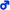## The Racing Forum Game Project

Need a bit of advice on something? Ask right here!Moderator: pinkteddyx64

TBAAJ Volunteer Team
Posts: 3346
Joined: 28 Nov 2015, 02:46
Reputation: 261
Gender:### The Racing Forum Game Project

Edit: Changed title for general info relating to my new project and stuff I may need help with

@Kyx @spotify95

Working on a racing forum game, bare with me. I'm stuck as I think I'm getting mistakes with my velocity formulas

1) If a car accelerates at 12.9199m/s^2 from rest/from zero how long does it take to reach 100 metres? And what speed will it be at at 100m?

Kyx
TBAAJ Volunteer Team
Posts: 1373
Joined: 02 May 2017, 15:18
Reputation: 481
Gender:Contact:

### Re: Maths help!

coleslaw wrote:@Kyx @spotify95

Working on a racing forum game, bare with me. I'm stuck as I think I'm getting mistakes with my velocity formulas

1) If a car accelerates at 12.9199m/s^2 from rest/from zero how long does it take to reach 100 metres? And what speed will it be at at 100m?

final speed = starting speed + [(acceleration) x (time)]
distance = [(starting speed) x (time)] + [(0.5) x (acceleration) x (time) x (time)]

100 meters = [ (0) x (time)] + [(0.5) x (12.9199) x (time) x (time)]
(time) squared = 100 / 12.9199 = 7.7399
time = square root (7.7399) = 2.7821 seconds

final speed = 0 + (12.9199) x (2.7821) = 35.9445 m/s

My website can be found here: http://quantumquasar.forumotion.com/
TBAAJ Volunteer Team
Posts: 3346
Joined: 28 Nov 2015, 02:46
Reputation: 261
Gender:### Re: Maths help!

Kyx wrote:
coleslaw wrote:@Kyx @spotify95

Working on a racing forum game, bare with me. I'm stuck as I think I'm getting mistakes with my velocity formulas

1) If a car accelerates at 12.9199m/s^2 from rest/from zero how long does it take to reach 100 metres? And what speed will it be at at 100m?

final speed = starting speed + [(acceleration) x (time)]
distance = [(starting speed) x (time)] + [(0.5) x (acceleration) x (time) x (time)]

100 meters = [ (0) x (time)] + [(0.5) x (12.9199) x (time) x (time)]
(time) squared = 100 / 12.9199 = 7.7399
time = square root (7.7399) = 2.7821 seconds

final speed = 0 + (12.9199) x (2.7821) = 35.9445 m/s

If 100m=0.5*12.9199*t^2 shouldn't it be 100/6.45995 not 100/12.9199?

I've got a feeling I've got my acceleration wrong. I derived it from some F1 data of a car that accelerates from 0-100Km/H in 2.15 seconds. I did 100,000/(2.15*3600) to get 12.9199m/s/s is that right?

TBAAJ Volunteer Team
Posts: 3346
Joined: 28 Nov 2015, 02:46
Reputation: 261
Gender:### Re: Maths help!

Ignore previous post I figured where I went wrong.
0-100KmH in 2.15 secs. 100KmH is 27.77778 m/s. This means an acceleration of 27.77778/2.15 = 12.9199m/s^2
Now accelerating from rest at 12.9199m/s^2 until 100m: 100 = 0 + 0.5*12.9199*t^2
100 = 6.45995*t^2
t^2 = 100/6.45995 = 15.48
t = 3.934462603101095

So final speed = (3.934462603101095)*12.9199 = 50.83286338580584m/s (Before I used the s=dt formula not accounting for accel.)

TBAAJ Volunteer Team
Posts: 3346
Joined: 28 Nov 2015, 02:46
Reputation: 261
Gender:### Re: The Racing Forum Game Project

@Kyx @pinkteddyx64 @spotify95 Are any of you good with Microsoft Excel?

With a final speed of 50.833m/s after 100m, this is equalivent to 183KmH
However, the car has three acceleration stats: From 0-100, 100-200 and 200-300KmH

I need to find the distance/point at which the car hits 100KmH as when it hits 100KmH its acceleration changes from 12.9199m/s^2 to 12.34568m/s^2
It hits 100KmH (27.777m/s) after 2.15secs so the distance is 0.5*(0+27.777)*2.15 = 29.86111111111111 metres, is this right?
So now we do 100m-29.861m=70.1388888888888m and the car accelerates this remaining distance at 12.34568m/s^2
So final speed = sqrt(27.777m/s^2 + 2*12.34568*70.138m) = 41.9m/s = 151KmH

So as we can see the original 183KmH compared to the 151KmH makes sense because the second one the car accelerates at a slightly slower pace after 29.8m so it the final speed would be slower.

are these calculations correct?

Kyx
TBAAJ Volunteer Team
Posts: 1373
Joined: 02 May 2017, 15:18
Reputation: 481
Gender:Contact:

### Re: The Racing Forum Game Project

coleslaw wrote:@Kyx @pinkteddyx64 @spotify95 Are any of you good with Microsoft Excel?

With a final speed of 50.833m/s after 100m, this is equalivent to 183KmH
However, the car has three acceleration stats: From 0-100, 100-200 and 200-300KmH

I need to find the distance/point at which the car hits 100KmH as when it hits 100KmH its acceleration changes from 12.9199m/s^2 to 12.34568m/s^2
It hits 100KmH (27.777m/s) after 2.15secs so the distance is 0.5*(0+27.777)*2.15 = 29.86111111111111 metres, is this right?
So now we do 100m-29.861m=70.1388888888888m and the car accelerates this remaining distance at 12.34568m/s^2
So final speed = sqrt(27.777m/s^2 + 2*12.34568*70.138m) = 41.9m/s = 151KmH

So as we can see the original 183KmH compared to the 151KmH makes sense because the second one the car accelerates at a slightly slower pace after 29.8m so it the final speed would be slower.

are these calculations correct?

Seems correct for the first one, havent tried the others yet

http://www.rg-s.co.uk/suvatequationcalc ... 9199&timt=

My website can be found here: http://quantumquasar.forumotion.com/